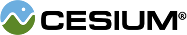#LinearSpline

#### new Cesium.LinearSpline(options) Core/LinearSpline.js 52

A spline that uses piecewise linear interpolation to create a curve.
Name Type Description
`options` Object Object with the following properties:
Name Type Description
`times` Array.<Number> An array of strictly increasing, unit-less, floating-point times at each point. The values are in no way connected to the clock time. They are the parameterization for the curve.
`points` Array.<Cartesian3> The array of `Cartesian3` control points.
##### Example:
``````var times = [ 0.0, 1.5, 3.0, 4.5, 6.0 ];
var spline = new Cesium.LinearSpline({
times : times,
points : [
new Cesium.Cartesian3(1235398.0, -4810983.0, 4146266.0),
new Cesium.Cartesian3(1372574.0, -5345182.0, 4606657.0),
new Cesium.Cartesian3(-757983.0, -5542796.0, 4514323.0),
new Cesium.Cartesian3(-2821260.0, -5248423.0, 4021290.0),
new Cesium.Cartesian3(-2539788.0, -4724797.0, 3620093.0)
]
});

var p0 = spline.evaluate(times);``````

### Members

#### readonlypoints : Array.<Cartesian3> Core/LinearSpline.js 99

An array of `Cartesian3` control points.

#### readonlytimes : Array.<Number> Core/LinearSpline.js 85

An array of times for the control points.

### Methods

#### evaluate(time, result) → Cartesian3 Core/LinearSpline.js 131

Evaluates the curve at a given time.
Name Type Description
`time` Number The time at which to evaluate the curve.
`result` Cartesian3 optional The object onto which to store the result.
##### Returns:
The modified result parameter or a new instance of the point on the curve at the given time.
##### Throws:
• DeveloperError : time must be in the range `[t0, tn]`, where `t0` is the first element in the array `times` and `tn` is the last element in the array `times`.

#### findTimeInterval(time) → Number Core/LinearSpline.js 118

Finds an index `i` in `times` such that the parameter `time` is in the interval `[times[i], times[i + 1]]`.
Name Type Description
`time` Number The time.
##### Returns:
The index for the element at the start of the interval.
##### Throws:
• DeveloperError : time must be in the range `[t0, tn]`, where `t0` is the first element in the array `times` and `tn` is the last element in the array `times`.